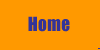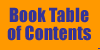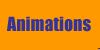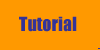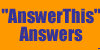Animations Fundamentals of Sound contains several figures that attempt to show how something, such as a longitudinal wave, changes in time, by a series of figures, each changed slightly from the previous. Examples are Figures 1-1 and 1-2. It is easier to see what is going on in time by watching the real thing, or at least a simulation of it. Such simulations, called animations, are what we present here. To see an animation associated with a figure in the book, click on the figure number below. Chapter 1: Figure 1-1.   Transverse wave motion. Figure 1-2.   Longitudinal wave motion. Figure 1-16. Reflection from a fixed end Figure 1-17. Reflection from a loose end. Figure 1-19. Constructive interference. Figure 1-20. Destructive interference.   Chapter 2: Figure 2-2. Formation of a standing wave. Chapter 4: A movie of the Tacoma Narrows bridge oscillations caused by resonance is available here. The bridge's vibration modes were excited by a strong wind. The bridge collapsed soon after the events shown here. Chapter 7: Figure 7-15. Motion of a square wave.   Chapter 10: Figure 10-6. Longitudinal pulse on a chain of masses and springs.   Chapter 12: Figure 12-10. Traveling wave on the basilar membrane.© William J. Mullin